Next: Pressure Force Up: Force Accumulation Previous: Gravity Force

## Spring Linear Force

After accumulation of external gravity force, we are moving into first internal body forces - linear spring force. Linearity means in that case that we derive expression directly from a Hooke's law 6 with a constant elasticity factor. Additional term with additional factor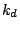is a damping term.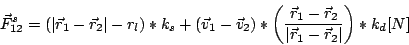(1)

where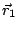is position of first spring point,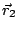is a position of second spring point,is rest length of the spring (length, where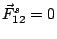) stored in the spring structure.andare spring and damping factors.
Implementation of linear spring accumulation procedure for one spring is straighforward. First we calculate distance between two points of the spring (start - end point) and check if it isthan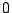. If distance is equal to- we simply skip calculation, since that distance gives us non continuality in the spring force expression. Then we compute difference of velocities which is needed for damping term calculation. After that all calculated quantities are collected to compute the force value. At the end - force value is multiplied by vector "from point 1 to point 2" to get force vector and added to force accumulator of first (1) and substracted from accelerator of second (2) point. A part of accumulation procedure, responsible for linear force calculation is presented below.

/* loop over all springs */
for(i=1 ; i <= NUMS ; ++i)
{
// get positions of spring start & end points
x1 = myRelPoints[ mySprings[i].i ].x;
y1 = myRelPoints[ mySprings[i].i ].y;
x2 = myRelPoints[ mySprings[i].j ].x;
y2 = myRelPoints[ mySprings[i].j ].y;

// calculate sqr(distance)
r12d = sqrt (
(x1 - x2) *(x1 - x2) +
(y1 - y2) * (y1 - y2) );

if(r12d != 0)    // start = end?
{

// get velocities of start & end points
vx12 = myRelPoints[ mySprings[i].i ].vx -
myRelPoints[ mySprings[i].j ].vx;

vy12 = myRelPoints[ mySprings[i].i ].vy -
myRelPoints[ mySprings[i].j ].vy;

// calculate force value
f = (r12d -  mySprings[i].length) * KS +
(vx12 * (x1 - x2) +
vy12 * (y1 - y2)) * KD / r12d;

// force vector
Fx = ((x1 - x2) / r12d ) * f;
Fy = ((y1 - y2) / r12d ) * f;

// accumulate force for starting point
myRelPoints[ mySprings[i].i  ].fx -= Fx;
myRelPoints[ mySprings[i].i  ].fy -= Fy;

// accumulate force for end point
myRelPoints[  mySprings[i].j  ].fx += Fx;
myRelPoints[  mySprings[i].j  ].fy += Fy;

}

// Calculate normal vectors to springs
mySprings[i].nx =  (y1 - y2) / r12d;
mySprings[i].ny =  -(x1 - x2) / r12d;
}


In above procedure not only spring force has been calculated. We compute also normal vectors to springs and store them in springs structures. Why we do that? It will be used further in procedure of pressure force calculation. When spring forces for all springs has been calculated we are ready to make last step of force accumulation procedure - to calculate pressure force. To this point all the procedures were some kind of standard for spring-mass models. From now I will describe something brand new - an implementation of specific type of force which appear only in pressure model of soft bodies. Please refer to  for details about physics background and derivation of that force.Next: Pressure Force Up: Force Accumulation Previous: Gravity Force
Maciej Matyka 2004-03-30